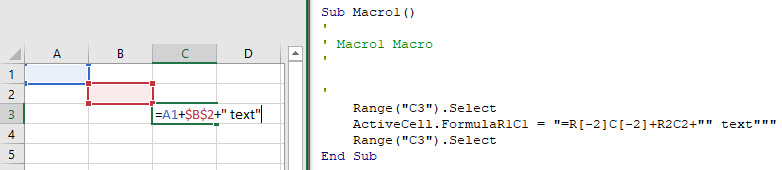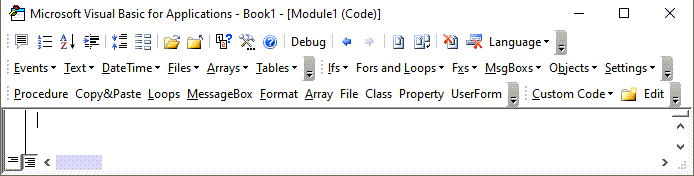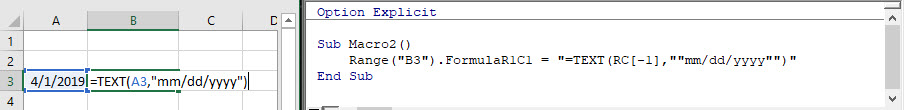# Excel VBA Formulas – The Ultimate Guide

This tutorial will teach you how to create cell formulas using VBA.

## Formulas in VBA

Using VBA, you can write formulas directly to Ranges or Cells in Excel. It looks like this:

``````Sub Formula_Example()

'Assign a hard-coded formula to a single cell
Range("b3").Formula = "=b1+b2"

'Assign a flexible formula to a range of cells
Range("d1:d100").FormulaR1C1 = "=RC2+RC3"

End Sub``````

There are two Range properties you will need to know:

• .Formula – Creates an exact formula (hard-coded cell references). Good for adding a formula to a single cell.
• .FormulaR1C1 – Creates a flexible formula. Good for adding formulas to a range of cells where cell references should change.

For simple formulas, it’s fine to use the .Formula Property.  However, for everything else, we recommend using the Macro Recorder

## Macro Recorder and Cell Formulas

The Macro Recorder is our go-to tool for writing cell formulas with VBA.  You can simply:

• Start recording
• Type the formula (with relative / absolute references as needed) into the cell & press enter
• Stop recording
• Open VBA and review the formula, adapting as needed and copying+pasting the code where needed.

I find it’s much easier to enter a formula into a cell than to type the corresponding formula in VBA.Notice a couple of things:

• The Macro Recorder will always use the .FormulaR1C1 property
• The Macro Recorder recognizes Absolute vs. Relative Cell References

## VBA FormulaR1C1 Property

The FormulaR1C1 property uses R1C1-style cell referencing (as opposed to the standard A1-style you are accustomed to seeing in Excel).

Here are some examples:

``````Sub FormulaR1C1_Examples()

'Reference D5 (Absolute)
'=\$D\$5
Range("a1").FormulaR1C1 = "=R5C4"

'Reference D5 (Relative) from cell A1
'=D5
Range("a1").FormulaR1C1 = "=RC"

'Reference D5 (Absolute Row, Relative Column) from cell A1
'=D\$5
Range("a1").FormulaR1C1 = "=R5C"

'Reference D5 (Relative Row, Absolute Column) from cell A1
'=\$D5
Range("a1").FormulaR1C1 = "=RC4"

End Sub``````

Notice that the R1C1-style cell referencing allows you to set absolute or relative references.

### Absolute References

In standard A1 notation an absolute reference looks like this: “=\$C\$2”.  In R1C1 notation it looks like this: “=R2C3”.

To create an Absolute cell reference using R1C1-style type:

• R + Row number
• C + Column number

Example:  R2C3 would represent cell \$C\$2 (C is the 3rd column).

``````    'Reference D5 (Absolute)
'=\$D\$5
Range("a1").FormulaR1C1 = "=R5C4"``````

### Relative References

Relative cell references are cell references that “move” when the formula is moved.

In standard A1 notation they look like this: “=C2”. In R1C1 notation, you use brackets [] to offset the cell reference from the current cell.

Example: Entering formula “=RC” in cell B3 would reference cell D4 (the cell 1 row below and 1 column to the right of the formula cell).

Use negative numbers to reference cells above or to the left of the current cell.

``````    'Reference D5 (Relative) from cell A1
'=D5
Range("a1").FormulaR1C1 = "=RC"``````

### Mixed References

Cell references can be partially relative and partially absolute.  Example:

``````    'Reference D5 (Relative Row, Absolute Column) from cell A1
'=\$D5
Range("a1").FormulaR1C1 = "=RC4"``````

Stop searching for VBA code online. Learn more about AutoMacro - A VBA Code Builder that allows beginners to code procedures from scratch with minimal coding knowledge and with many time-saving features for all users!## VBA Formula Property

When setting formulas with the .Formula Property you will always use A1-style notation.  You enter the formula just like you would in an Excel cell, except surrounded by quotations:

``````    'Assign a hard-coded formula to a single cell
Range("b3").Formula = "=b1+b2"``````

## VBA Formula Tips

### Formula With Variable

When working with Formulas in VBA, it’s very common to want to use variables within the cell formulas.  To use variables, you use & to combine the variables with the rest of the formula string. Example:

``````Sub Formula_Variable()
Dim colNum As Long
colNum = 4

Range("a1").FormulaR1C1 = "=R1C" & colNum & "+R2C" & colNum

End Sub``````

VBA Programming | Code Generator does work for you!

### Formula Quotations

If you need to add a quotation (“) within a formula, enter the quotation twice (“”):``````Sub Macro2()
Range("B3").FormulaR1C1 = "=TEXT(RC[-1],""mm/dd/yyyy"")"
End Sub``````

A single quotation (“) signifies to VBA the end of a string of text. Whereas a double quotation (“”) is treated like a quotation within the string of text.

Similarly, use 3 quotation marks (“””) to surround a string with a quotation mark (“)

``````MsgBox """Use 3 to surround a string with quotes"""
' This will print <"Use 3 to surround a string with quotes"> immediate window``````

### Assign Cell Formula to String Variable

We can read the formula in a given cell or range and assign it to a string variable:

``````'Assign Cell Formula to Variable
Dim strFormula as String
strFormula = Range("B1").Formula``````

### Different Ways to Add Formulas to a Cell

Here are a few more examples for how to assign a formula to a cell:

1. Directly Assign Formula
2. Define a String Variable Containing the Formula
3. Use Variables to Create Formula
``````Sub MoreFormulaExamples ()
' Alternate ways to add SUM formula
' to cell B1
'
Dim strFormula as String
Dim cell as Range
dim fromRow as long, toRow as long

Set cell = Range("B1")

' Directly assigning a String
cell.Formula = "=SUM(A1:A10)"

' Storing string to a variable
' and assigning to "Formula" property
strFormula = "=SUM(A1:A10)"
cell.Formula = strFormula

' Using variables to build a string
' and assigning it to "Formula" property
fromRow = 1
toRow   = 10
strFormula = "=SUM(A" & fromValue & ":A" & toValue & ")
cell.Formula = strFormula
End Sub``````

### Refresh Formulas

As a reminder, to refresh formulas, you can use the Calculate command:

``Calculate``

To refresh single formula, range, or entire worksheet use .Calculate instead:

``Sheets("Sheet1").Range("a1:a10").Calculate``